# animatplot¶

version: 0.4.1 Github

animatplot is a library for producing interactive animated plots in python built on top of matplotlib.Contents

## Installation¶

Using conda:

conda install animatplot -c conda-forge


Using pip:

pip install animatplot


Warning

If matplotlib was installed with anaconda, please upgrade matplotlib to >= 2.2 with anaconda before installing animatplot with pip. Otherwise, pip may butcher your environment(s).

If you are using jupyter lab, then install jupyter-matplotlib.

## Tutorial¶

### Getting Started¶

Animatplot is built on the concept of blocks. We’ll start by animating a Line block.

First we need some imports.

Note

Interactivity is not available in the static docs. Run the code locally to get interactivity.

#### Basic Animation¶

:

%matplotlib notebook
import numpy as np
import matplotlib.pyplot as plt
import animatplot as amp


We will animate the function:

$$y = \sin(2\pi(x+t))$$ over the range $$x=[0,1]$$, and $$t=[0,1]$$

Let’s generate the data:

:

x = np.linspace(0, 1, 50)
t = np.linspace(0, 1, 20)

X, T = np.meshgrid(x, t)
Y = np.sin(2*np.pi*(X+T))


In order to tell animatplot how to animate the data, we must pass it into a block. By default, the Line block will consider each of the rows in a 2D array to be a line at a different point in time.

We then pass a list of all our blocks into an Animation, and show the animation.

:

block = amp.blocks.Line(X, Y)
anim = amp.Animation([block])

anim.save_gif('images/line1') # save animation for docs
plt.show()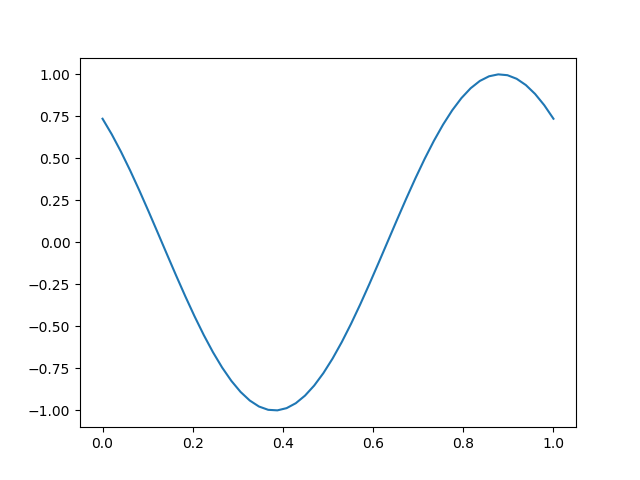#### Adding Interactivity¶

We’ll use the same data to make a new animation with interactive controls.

:

block = amp.blocks.Line(X, Y)
anim = amp.Animation([block])

anim.controls() # creates a timeline_slider and a play/pause toggle
anim.save_gif('images/line2') # save animation for docs
plt.show()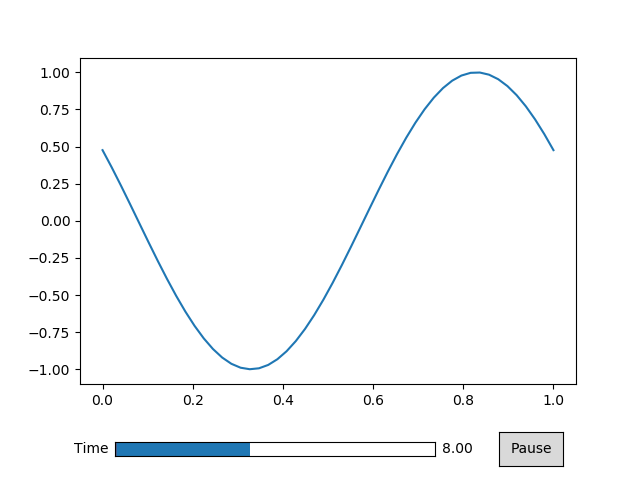#### Displaying the Time¶

The above animation didn’t display the time properly because we didn’t tell animatplot what the values of time are. Instead it displayed the frame number. We can simply pass our values of time into our call to Animation.

:

block = amp.blocks.Line(X, Y)
anim = amp.Animation([block], t) # pass in the time values

anim.controls()
anim.save_gif('images/line3') # save animation for docs
plt.show()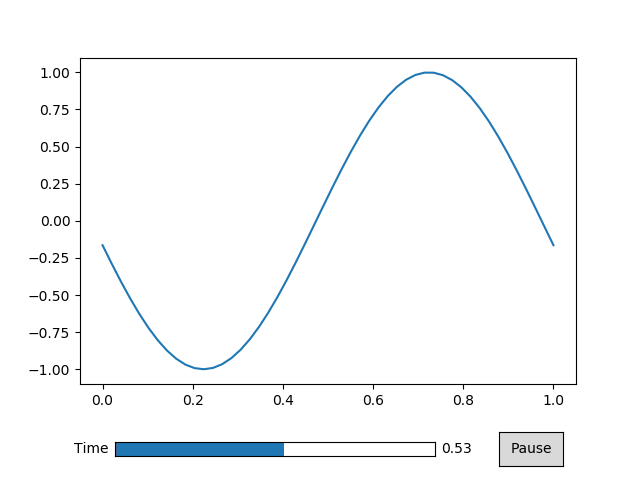#### Controlling Time¶

Simply passing in the values of time into the call to Animation doesn’t give us much control. Instead we use a Timeline.

:

timeline = amp.Timeline(t, units='s', fps=20)


The units argument will set text to be displayed next to the time number.

The fps argument gives you control over how fast the animation will play.

:

block = amp.blocks.Line(X, Y)
anim = amp.Animation([block], timeline) # pass in the timeline instead

anim.controls()
anim.save_gif('images/line4') # save animation for docs
plt.show()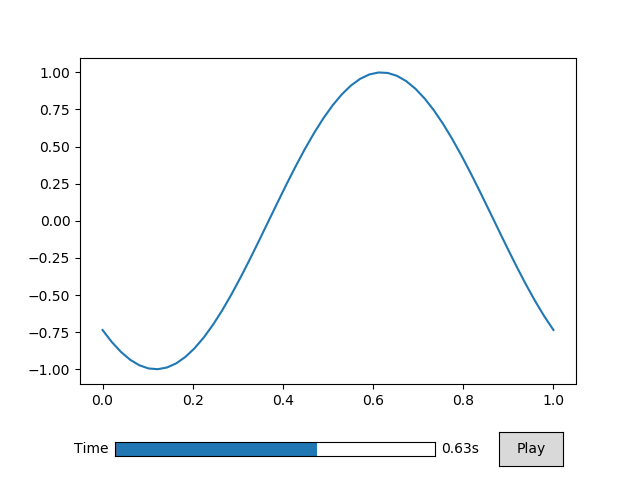#### Built on Matplotlib¶

Since animatplot is build on matplotlib, we can use all of our matplotlib tools.

:

block = amp.blocks.Line(X, Y, marker='.', linestyle='-', color='r')
anim = amp.Animation([block], timeline)

# standard matplotlib stuff
plt.title('Sine Wave')
plt.xlabel('x')
plt.ylabel('y')

anim.controls()
anim.save_gif('images/line5') # save animation for docs
plt.show()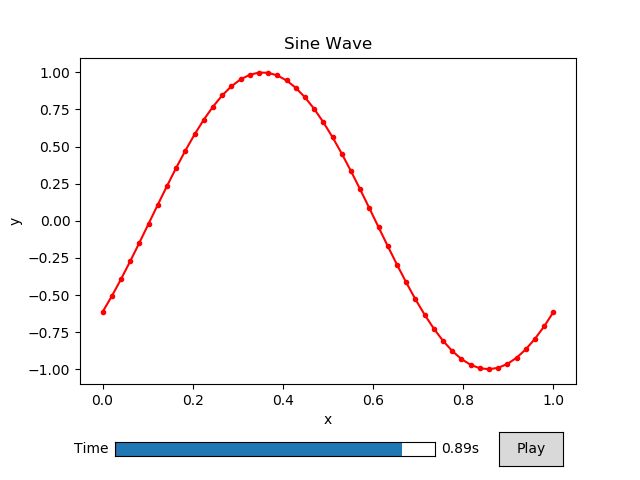### Using multiple blocks¶

Here we are going to use 2 different blocks in our animation.

First we need some imports:

:

%matplotlib notebook
import numpy as np
import matplotlib.pyplot as plt
import animatplot as amp


We are going to plot a pcolormesh and a line on 2 different axes.

Let’s use: $$z = \sin(x^2+y^2-t)$$ for the pcolormesh, and a cross-section of $$y=0$$: $$z = \sin(x^2-t)$$ for the line.

First, we generate the data.

:

x = np.linspace(-2, 2, 41)
y = np.linspace(-2, 2, 41)
t = np.linspace(0, 2*np.pi, 30)

X, Y, T = np.meshgrid(x, y, t)

pcolormesh_data = np.sin(X*X+Y*Y-T)
line_data       = pcolormesh_data[20,:,:] # the slice where y=0


We need to be careful here. Our time axis is the last axis of our data, but animatplot assumes it is the first axis by default. Fortunately, we can use the t_axis argument.

We use the ax argument to attached the data to a specific subplot.

:

# standard matplotlib stuff
# create the different plotting axes
fig, (ax1, ax2) = plt.subplots(1, 2)

for ax in [ax1, ax2]:
ax.set_aspect('equal')
ax.set_xlabel('x')

ax2.set_ylabel('y', labelpad=-5)
ax1.set_ylabel('z')
ax1.set_ylim([-1.1,1.1])

fig.suptitle('Multiple blocks')
ax1.set_title('Cross Section: $y=0$')
ax2.set_title(r'$z=\sin(x^2+y^2-t)$')

# animatplot stuff
# now we make our blocks
line_block       = amp.blocks.Line(X[0,:,:], line_data,
ax=ax1, t_axis=1)
pcolormesh_block = amp.blocks.Pcolormesh(X[:,:,0], Y[:,:,0], pcolormesh_data,
ax=ax2, t_axis=2, vmin=-1, vmax=1)
plt.colorbar(pcolormesh_block.quad)
timeline = amp.Timeline(t, fps=10)

# now to contruct the animation
anim = amp.Animation([pcolormesh_block, line_block], timeline)
anim.controls()

anim.save_gif('images/multiblock')
plt.show()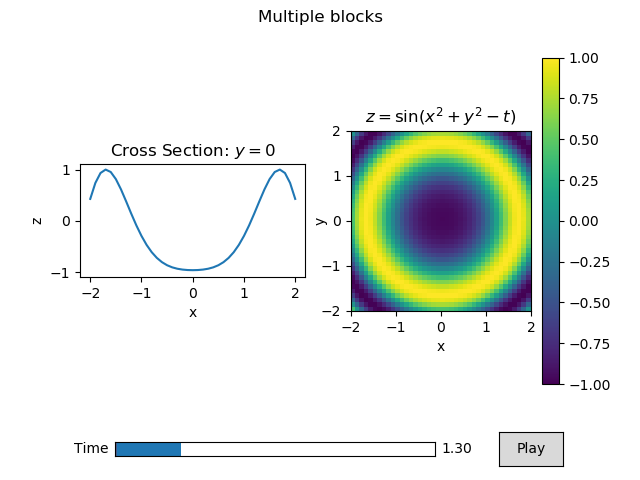There is a lot going on here so lets break it down.

Firstly, the standard matplotlib stuff is creating, and labeling all of our axes for our subplot. This is exactly how one might do a static, non-animated plot.

When we make the Line block, we pass in the data for our lines as 2D arrays (X[0,:,:] and line_data). We attached that line to the first matplotlib axes ax=ax1. We also specifify that the time axis is the last axis of the data t_axis=1.

When we make the Pcolormesh block, we pass in the x, y data as 2D arrays (X[:,:,0] and Y[:,:,0]), and the z data as a 3D array. We attached the pcolormesh to the second matplotlib axes ax=ax2. We also specifify that the time axis is the last axis of the data t_axis=2.

Additional, we told the Pcolormesh blocks what the minimum and maximum values will be (vmin=-1 and vmax=1), so that the colorscale will be proper. The keywords vmin, and vmax get passed to the underlaying called to matplotlib’s pcolormesh.

plt.colorbar does not recognize the Pcolormesh block as a mappable, so we pass in a mappable from the block to get the colorbar to work. In the future, animatplot may have a wrapper around this.

The rest simply brings all of the blocks, and the timeline together into an animation.### Custimizing the Controls¶

Here we’ll like how to manipulate the timeline_slider and the toggle button.

The interactive controls can be make using the controls() method of the animation class, as in the getting started tutorial, but this method is a wrapper around the toggle and timeline_slider methods.

First, we need from imports and data to animate.

:

%matplotlib notebook
import numpy as np
import matplotlib.pyplot as plt
import animatplot as amp

:

x = np.linspace(0, 1, 50)
t = np.linspace(0, 1, 20)

X, T = np.meshgrid(x, t)
Y = np.sin(2*np.pi*(X+T))

Animation.toggle(ax=None)[source]

Creates a play/pause button to start/stop the animation

Parameters: ax (matplotlib.axes.Axes, optional) – The matplotlib axes to attach the button to.
Animation.timeline_slider(text=’Time’, ax=None, valfmt=None, color=None)[source]

Creates a timeline slider.

Parameters: text (str, optional) – The text to display for the slider. Defaults to ‘Time’ ax (matplotlib.axes.Axes, optional) – The matplotlib axes to attach the slider to. valfmt (str, optional) – a format specifier used to print the time Defaults to ‘%s’ for datetime64, timedelta64 and ‘%1.2f’ otherwise. color – The color of the slider.
Animation.controls(timeline_slider_args={}, toggle_args={})[source]

Creates interactive controls for the animation

Creates both a play/pause button, and a time slider at once

Parameters: timeline_slider_args (Dict, optional) – A dictionary of arguments to be passed to timeline_slider() toggle_args (Dict, optional) – A dictionary of argyments to be passed to toggle()

Now to make the animation

By specifying the ax parameter, we can change the position of either the toggle or the timeline_slider.

We use color to change the color of the slider, and valfmt to change how the time is displayed.

Let’s create our block, then create the controls at the top of the animation.

:

block = amp.blocks.Line(X, Y)

plt.subplots_adjust(top=0.8) # squish the plot to make space for the controls
slider_ax = plt.axes([.18, .89, .5, .03]) # the rect of the axis
button_ax = plt.axes([.78, .87, .1, .07]) # x, y, width, height

anim = amp.Animation([block])

anim.toggle(ax=button_ax)
anim.timeline_slider(text='TIME', ax=slider_ax, color='red', valfmt='%1.0f')
# equivalent to:
# anim.controls({'text':'TIME', 'ax':slider_ax, 'color':'red', 'valfmt':'%1.0f'},
#               {'ax':button_axis})

anim.save_gif('images/controls')
plt.show()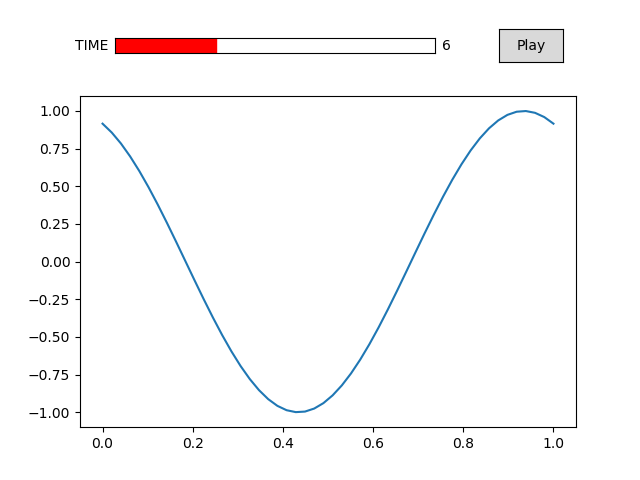### Using Jupyter¶

In order to display interactive animations in jupyter notebook or lab, use one of the following line magics:

%matplotlib notebook  # notebook only
%matplotlib ipympl  # notebook or lab
%matplotlib widget  # notebook or lab (equivalent to ipympl)


Or if you prefer matplotlib to display a figure in a native window:

%matplotlib qt


## API¶

Animatplot is build on top of three main classes:

• Animation
• Block
• Timeline

A Timeline holds the information and logic to actually control the timing of all animations.

A Block represents any “thing” that is to be animated.

An Animation is a composition of a list of blocks and a timeline. This class builds the final animation.

### Animation¶

 Animation(blocks[, timeline, fig]) The foundation of all animations.

### Timeline¶

 Timeline(t[, units, fps, log]) An object to contain and control all of the time

### blocks¶

Blocks handle the animation of different types of data. The following blocks are available in animatplot.blocks.

#### Data blocks¶

These blocks are built to animate data.

 Line(*args[, ax, t_axis]) Animates a single line. Quiver(X, Y, U, V[, ax, t_axis]) A block for animated quiver plots Pcolormesh(*args[, ax, t_axis]) Animates a pcolormesh Imshow(images[, ax, t_axis]) Animates a series of images Scatter(x, y[, s, c, ax, t_axis]) Animates scatter plots

#### Graph Label Blocks¶

These blocks are for animating non-data features on an axis.

 Title(text[, ax]) Animates an axes title.

#### Advanced Blocks¶

These blocks are for more advanced use. These allow you to write more custom animations.

 Block([ax, t_axis]) A base class for blocks Update(func, length[, fargs, ax]) For providing a custom update method Nuke(func, length[, fargs, ax]) For when the other blocks just won’t do

#### Composition Blocks¶

These blocks are amalgamations of other blocks. These are actually functions that return lists of blocks.

 vector_comp(X, Y, U, V[, skip, t_axis, …]) produces an animation of vector fields

### Animatplot.animations¶

The animations subpackage is an opinionated subpackage that contains a number of convenience functions for specific use cases. These functions wrap around different combinations of blocks.

In general, these functions will take some data to be animated, some parameters to tell animatplot what to do, then dictionaries of parameters to pass to the underlying blocks.

Warning

This subpackage is less API stable than the above (blocks/Animation/Timeline), and some of these functions may have different defaults.

This submodule contains the following functions.

 vector_plot(X, Y, U, V, t[, skip, t_axis, …]) produces an animation of vector fields

## Developer Setup¶

### Requirements¶

The following are required to build the docs.

sphinx
ipykernel
nbsphinx
matplotlib>=2.2
sphinx_rtd_theme
numpy


### Install¶

Clone and install the repository:

git clone https://github.com/t-makaro/animatplot.git
cd animatplot
pip install -e .


### Testing¶

From the root animatplot directory simply run:

pytest


Warning

Tests are currently very limited. Please run examples to ensure everything works.

### Linting¶

This project currently uses pycodestyle for linting.

## Changes to animatplot¶

### 0.4.0¶

Features

• New Title block. #27
• x values are now optional in the Line Block. #29

Bug Fixes

• Timeline slider now formats np.datetime properly by default. #21

Breaking Changes

• The x, y inputs to the Line Block are not positional only. #29
• Removed axis kwargs that deprecated in favor of ax in 0.3.0. #31

Developer Changes

• Testing tools were moving into the tests folder and are no longer a subpackage of animatplot. #26

Authors

* indicates new author in this release.

### 0.3.0¶

Deprecations

• The axis keyword argument has been replaced (everywhere) in favour of ax, and axis will be removed completely in 0.4.0. This does not apply to t_axis which is unchanged. See #10 for rational.

Features

• The Pcolormesh block now accepts 1D arrays (in addition to 2D) for x and y inputs.

• Animation.timeline_slider now accepts a text argument to change the name of the slider.

• New blocks:

• Scatter for animating scatter plots. Capable of animating size and position of the points, but not yet the color.
• Update a block that accepts a generic function that takes a frame number. Good if another block doesn’t already exist for some tasks.
• Composition Blocks: These are functions that return a list of blocks (and maybe a timeline). These are in the blocks subpackage and can be identified by the _comp suffix.

• New (and very experimental) animations subpackage (well new to the public api). Contains some new convenice functions.

• vector_plot wraps Pcolormesh and Quiver to produce animated vector fields.

Bug Fixes

• Previously, an Animation with a timeline_slider, but no toggle would cause an error.

Breaking Changes

• The text argument to timeline_slider is now the first positional argument.
• The order of positional arguments for the Nuke block has changed. This was required to give the ax argument a default.

Developer Changes

• New animation unittesting framework
• Tests / doc building now runs on CircleCI.

### 0.2.2¶

• Fix .animations and .blocks subpackages not being distributed properly.

### 0.2.0¶

• Complete and total overhaul of animatplot using with the idea of blocks as a foundation
• Chuck all previous attempts to support python 2 in the dumpster

### 0.1.0.dev3¶

This is the original release.